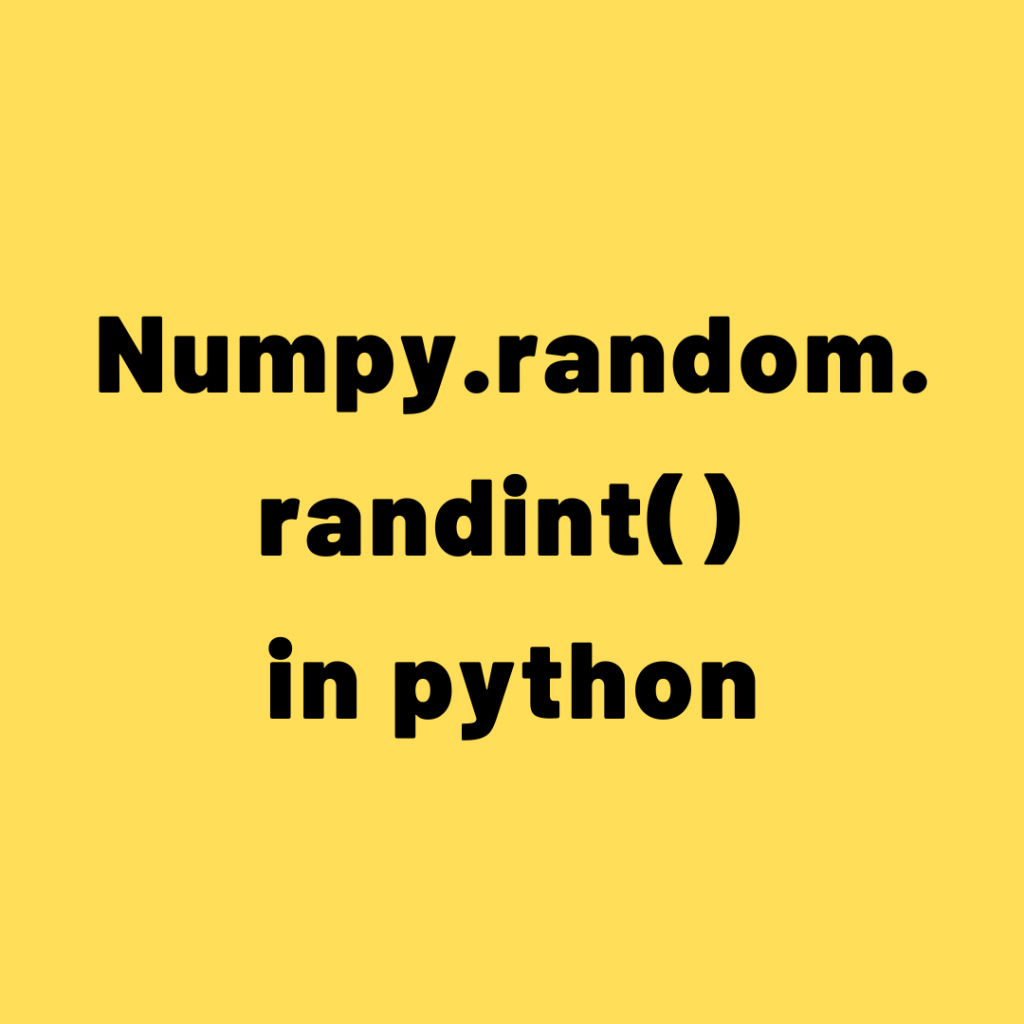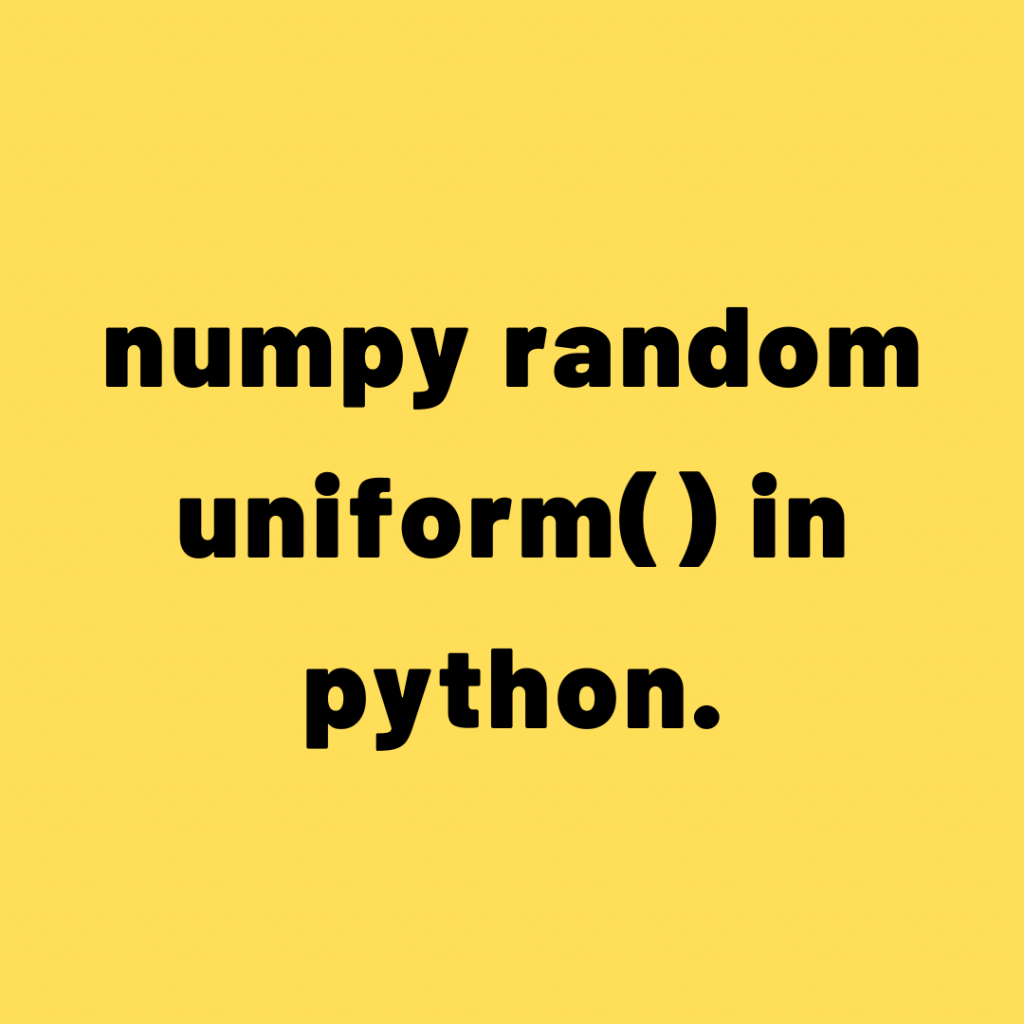Drop a Query

# Numpy## Numpy.random.randint() in python

numpy.random.randint function is used to get random integers from low to high values. The low value is included while the high value is excluded in the calculations. The output values are taken from the discrete uniform distribution of the range values. random.randint(low, high=None, size=None, dtype=int) Purpose: The numpy random randint function used for creating a …## How to use numpy.random.uniform() in python.

The np.random.uniform() function is used to create an array with random samples from a uniform probability distribution of given low and high values. random.uniform(low=0.0, high=1.0, size=None) Purpose: The numpy random uniform function used for creating a numpy array with random float values from low to high interval. Parameteres: Low: float or array-like of floats,optional: Lowest …## Numpy.sort() in python

The np.sort() function is used to sort the array along a specified axis. Numpy.sort (a, axis=- 1, kind=None, order=None) Purpose: This function is used for sorting the array. Parameters: arr:a:array_like array to be sorted. axis: None or int,optional Axis on which we perform the arithmetic mean if specified. otherwise, the arr will be flattened. kind: …## How to Use Numpy Where Function?

The numpy.where() function is used to filter data based on the conditions provided. These conditions can vary from being as simple as value comparisons to nested bit-wise conditions. You can also use this function to perform conditional replacements in the input data array. In this article, you will learn about the possible use cases of …## How to use Numpy Random Function in Python

How to use numpy.random.rand() function ? numpy.random.rand() function is used to generate random float values from an uniform distribution over [0,1). These values can be extracted as a single value or in arrays of any dimension. In this article, you will learn about various use cases of this function. Structural overview of numpy.random.rand() Syntax: numpy.random.rand(d0, …## Numpy Tutorial Part 2 – Vital Functions for Data Analysis

Numpy is the core package for data analysis and scientific computing in python. This is part 2 of a mega numpy tutorial. In this part, I go into the details of the advanced features of numpy that are essential for data analysis and manipulations. Introduction How to get index locations that satisfy a given condition …## Numpy Tutorial – Your first numpy guide to build python coding foundations

This is part 1 of the numpy tutorial covering all the core aspects of performing data manipulation and analysis with numpy’s ndarrays. Numpy is the most basic and a powerful package for scientific computing and data manipulation in python. Numpy Tutorial Part 1: Introduction to Arrays. Photo by Bryce Canyon. Also Read: Numpy Tutorial – …Course Preview

## Machine Learning A-Z™: Hands-On Python & R In Data Science

### Free Sample Videos:#### Machine Learning A-Z™: Hands-On Python & R In Data Science#### Machine Learning A-Z™: Hands-On Python & R In Data Science#### Machine Learning A-Z™: Hands-On Python & R In Data Science#### Machine Learning A-Z™: Hands-On Python & R In Data Science